# Calculus 2 : Polar Form

## Example Questions

1 2 3 4 5 6 7 8 10 Next →

### Example Question #16 : Polar Form

Calculate the polar form hypotenuse of the following cartesian equation: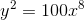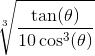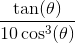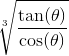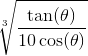Explanation:

In a cartesian form, the primary parameters areand. In polar form, they areand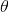is the hypotenuse, andis the angle created by.

2 things to know when converting from Cartesian to polar.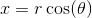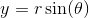You want to calculate the hypotenuse,Solution: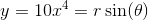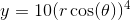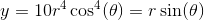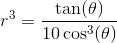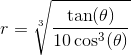### Example Question #259 : Parametric, Polar, And Vector

What is the polar form of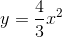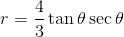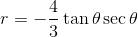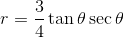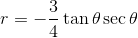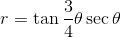Explanation:

We can convert from rectangular to polar form by using the following trigonometric identities: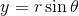and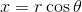. Given, then: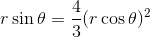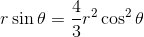Dividing both sides by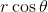, we get: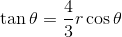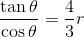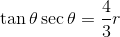### Example Question #91 : Polar Form

What is the polar form of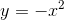?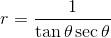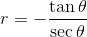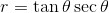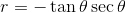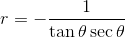Explanation:

We can convert from rectangular to polar form by using the following trigonometric identities:and. Given, then: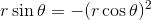Dividing both sides by, we get: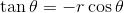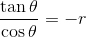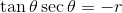### Example Question #92 : Polar Form

What is the polar form of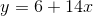?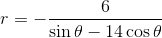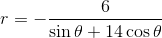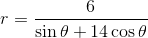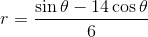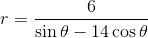Explanation:

We can convert from rectangular to polar form by using the following trigonometric identities:and. Given, then: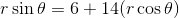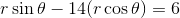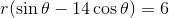### Example Question #93 : Polar Form

What is the polar form of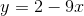?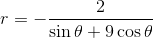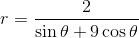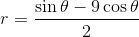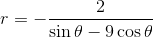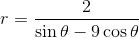Explanation:

We can convert from rectangular to polar form by using the following trigonometric identities:and. Given, then: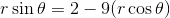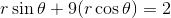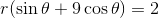1 2 3 4 5 6 7 8 10 Next →